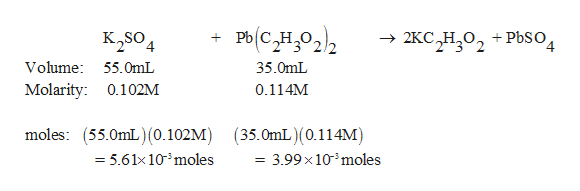# A 55.0 mL sample of 0.102M potassium sulfate solution is mixed with 35.0 mL of a 0.114M lead(II) acetate solution and the precipitation reaction shown here occurs:K2SO4(aq)+Pb(C2H3O2)2(aq)=2KC2H3O2(aq)+PbSO4(s).Determine the limiting reagent.

Question
120 views

A 55.0 mL sample of 0.102M potassium sulfate solution is mixed with 35.0 mL of a 0.114M lead(II) acetate solution and the precipitation reaction shown here occurs:

K2SO4(aq)+Pb(C2H3O2)2(aq)=2KC2H3O2(aq)+PbSO4(s).

Determine the limiting reagent.

check_circle

star
star
star
star
star
1 Rating
Step 1

The given balanced equation is given along wit...help_outlineImage TranscriptionclosePh(CH022 K2SO4 2KC H O +PbSO, 2 3 4 Volume: 55. OmL 35.0mL Molarity: 0.102M 0.114M (55.0mL) (0.102M) (35.0mL)(0.114M) = 3.99 x 10' moles moles: = 5.61x10'moles - fullscreen

### Want to see the full answer?

See Solution

#### Want to see this answer and more?

Solutions are written by subject experts who are available 24/7. Questions are typically answered within 1 hour.*

See Solution
*Response times may vary by subject and question.
Tagged in

### General Chemistry